# Low-Rank Approximation Toolbox: Randomized SVD

The randomized SVD and its relatives are workhorse algorithms for low-rank approximation. In this post, I hope to provide a fairly brief introduction to this useful method. In the following post, we’ll look into its analysis.

The randomized SVD is dead-simple to state: To approximate an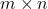matrix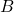by a rank-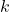matrix, perform the following steps:

1. Collect information. Form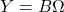where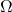is an appropriately chosen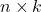random matrix.
2. Orthogonalize. Compute an orthonormal basis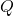for the column space of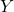by, e.g., thin QR factorization.
3. Project. Form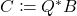. (Here,denotes the conjugate transpose of a complex matrix, which reduces to the ordinary transpose if the matrix is real.)

If all one cares about is a low-rank approximation to the matrix, one can stop the randomized SVD here, having obtained the approximation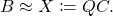As the name “randomized SVD” suggests, one can easily “upgrade” the approximation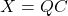to a compact SVD format:

1. SVD. Compute a compact SVD of the matrix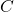: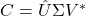where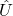and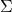and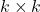and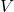is.
2. Clean up. Set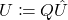.

We now have approximatedby a rank-matrix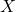in compact SVD form: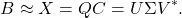One can use the factors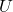,, andof the approximation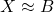as estimates of the singular vectors and values of the matrix.

## What Can You Do with the Randomized SVD?

The randomized SVD has many uses. Pretty much anywhere you would ordinarily use an SVD is a candidate for the randomized SVD. Applications include model reduction, fast direct solvers, computational biology, uncertainty quantification, among numerous others.

To speak in broad generalities, there are two ways to use the randomized SVD:

• As an approximation. First, we could useas a compressed approximation to. Using the format,requires just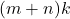numbers of storage, whereasrequires a much largernumbers of storage. As I detailed at length in my blog post on low-rank matrices, many operations are cheap for the low-rank matrix. For instance, we can compute a matrix–vector product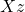in roughlyoperations rather than theoperations to compute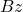. For these use cases, we don’t need the “SVD” part of the randomized SVD.
• As an approximate SVD. Second, we can actually use the,, andproduced by the randomized SVD as approximations to the singular vectors and values of. In these applications, we should be careful. Just becauseis a good approximation to, it is not necessarily the case that,, andare close to the singular vectors and values of. To use the randomized SVD in this context, it is safest to use posterior diagnostics (such as the ones developed in this paper of mine) to ensure that the singular values/vectors of interest are computed to a high enough accuracy. A useful rule of thumb is the smallest two to five singular values and vectors computed by the randomized SVD are suspect and should be used in applications only with extreme caution. When the appropriate care is taken and for certain problems, the randomized SVD can provide accurate singular vectors far faster than direct methods.

## Accuracy of the Randomized SVD

How accurate is the approximationproduced by the randomized SVD? No rank-approximation can be more accurate than the best rank-approximation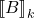to the matrix, furnished by an exact SVD oftruncated to rank. Thus, we’re interested in how much larger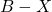is than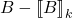.

### Frobenius Norm Error

A representative result describing the error of the randomized SVD is the following:

(1)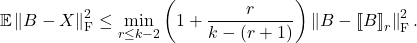This result states that the average squared Frobenius-norm error of the randomized SVD is comparable with the best rank-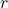approximation error for any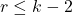. In particular, choosing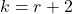, we see that the randomized SVD error is at most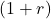times the best rank-approximation

(2)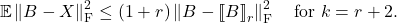Choosing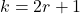, we see that the randomized SVD has at most twice the error of the best rank-approximation

(3)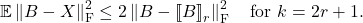In effect, these results tell us that if we want an approximation that is nearly as good as the best rank-approximation, using an approximation rank of, say,or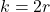for the randomized SVD suffices. These results hold even for worst-case matrices. For nice matrices with steadily decaying singular values, the randomized SVD can perform even better than equations (2)–(3) would suggest.

### Spectral Norm Error

The spectral norm is often a better error metric for matrix computations than the Frobenius norm. Is the randomized SVD also comparable with the best rank-approximation when we use the spectral norm? In this setting, a representative error bound is

(4)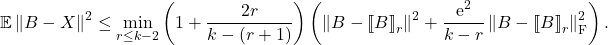The spectral norm error of the randomized SVD depends on the Frobenius norm error of the best rank-approximation for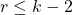.

Recall that the spectral and Frobenius norm can be defined in terms of the singular values of the matrix: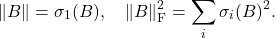Rewriting (4) using these formulas, we get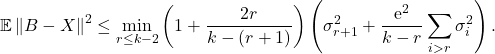Despite being interested in the largest singular value of the error matrix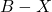, this bound demonstrates that the randomized SVD incurs errors based on the entire tail of‘s singular values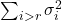. The randomized SVD is much worse than the best rank-approximation for a matrixwith a long detail of slowly declining singular values.

## Improving the Approximation: Subspace Iteration and Block Krylov Iteration

We saw that the randomized SVD produces nearly optimal low-rank approximations when we measure using the Frobenius norm. When we measure using the spectral norm, we have a split decision: If the singular values decay rapidly, the randomized SVD is near-optimal; if the singular values decay more slowly, the randomized SVD can suffer from large errors.

Fortunately, there are two fixes to improve the randomized SVD in the presence of slowly decaying singular values:

• Subspace iteration: To improve the quality of the randomized SVD, we can combine it with the famous power method for eigenvalue computations. Instead of, we use the powered expression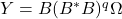. Powering has the effect of amplifying the large singular values ofand dimishing the influence of the tail.
• Block Krylov iteration: Subspace iteration is effective, but fairly wasteful. To compute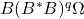we compute the block Krylov sequence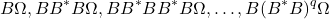and throw away all but the last term. To make more economical use of resources, we can use the entire sequence as our information matrix: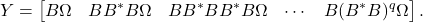Storing the entire block Krylov sequence is more memory-intensive but makes more efficient use of matrix products than subspace iteration.

Both subspace iteration and block Krylov iteration should be carefully implemented to produce accurate results.

Both subspace iteration and block Krylov iteration diminish the effect of a slowly decaying tail of singular values on the accuracy of the randomized SVD. The more slowly the tail decays, the larger one must take the number of iterations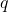to obtain accurate results.